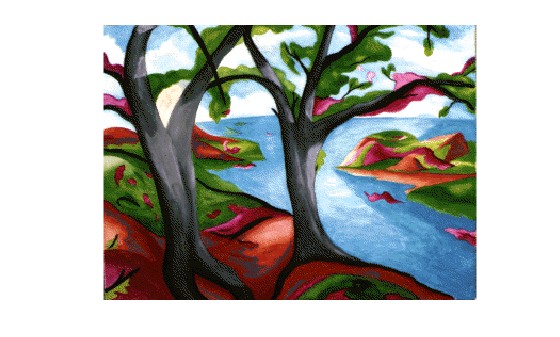Documentation

# roicolor

Select region of interest (ROI) based on color

## Syntax

``BW = roicolor(I,low,high)``
``BW = roicolor(I,v)``

## Description

example

````BW = roicolor(I,low,high)` returns an ROI selected as those pixels in image `I` that lie within the range [`low high`]. The returned value, `BW`, is a binary image with `0`s outside the region of interest and `1`s inside.```
````BW = roicolor(I,v)` returns an ROI selected as those pixels in image `I` that match values in vector `v`.```

## Examples

collapse all

Load an indexed image `X` wth color map `map`. The color map has 128 colors. Display the indexed image.

```load trees imshow(X,map)```Create a binary mask image based on color. The mask is `true` for pixels with index in the range [10, 20]. The mask is `false` for pixels with index outside this range.

`BW = roicolor(X,10,20);`

`imshow(BW)`## Input Arguments

collapse all

Indexed or grayscale image, specified as an m-by-n numeric matrix.

Minimum value to include in the ROI, specified as a numeric scalar.

Minimum value to include in the ROI, specified as a numeric scalar.

Set of values to include in the ROI, specified as a numeric vector.

## Output Arguments

collapse all

Binary image, returned as an m-by-n logical matrix.

Data Types: `logical`

## Tips

• You can use the returned image as a mask for masked filtering using `roifilt2`.

• If you specify a colormap range, ```[low high]```, then

`BW = (I >= low) & (I <= high)`
• If you specify a set of colormap values, `v`, then the mask generated by `roicolor` is equivalent to:

`BW = ismember(I,v)`